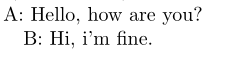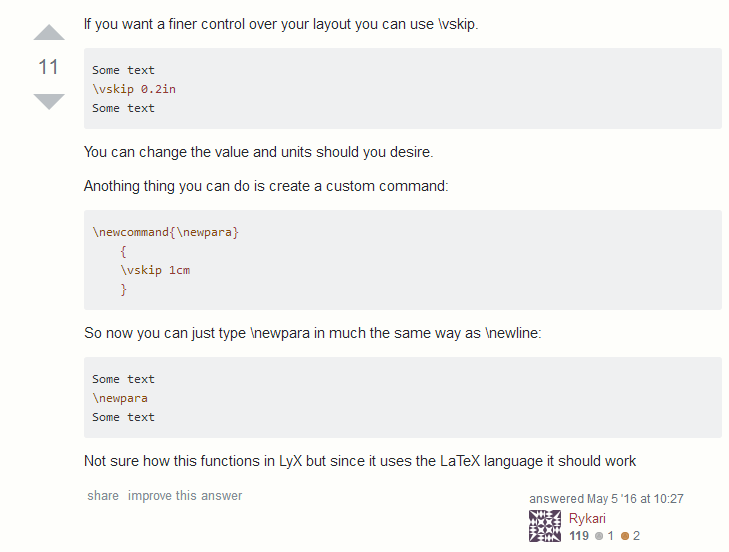WinEdt（latex）教程详细笔记

1.字体

字体大小

1 设置latex/winedt代码中字体大小
2 设置文章字体大小


\tiny
\scriptsize
\footnotesize
\small
\normalsize
\large
\Large
\LARGE
\huge
\Huge


{\small Hello word}


字体加粗

\textbf{这里放文本}


{\textbf{\small Hello word}}


$\boldsymbol 直接接数学符号$


3.空格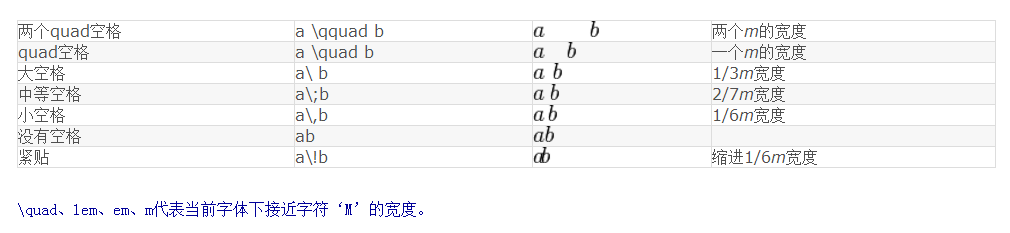$a$, $b$, $c$.

$a,\ b,\ c.$

The first three letter are a, b, c.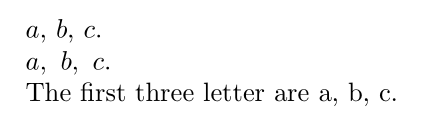5.数学模式与文本模式(包括行内公式和行间公式)

% !Mode::"TeX:UTF-8"
\documentclass{article}
\usepackage{amssymb}
\begin{document}
\section{symbol}
1+1=2, $$1+1=2$$, $I know 1+1=2,$

This is in text mode, $This is in math mode,$ $This\ is\ in\ math\ in\ mode.$

\end{document}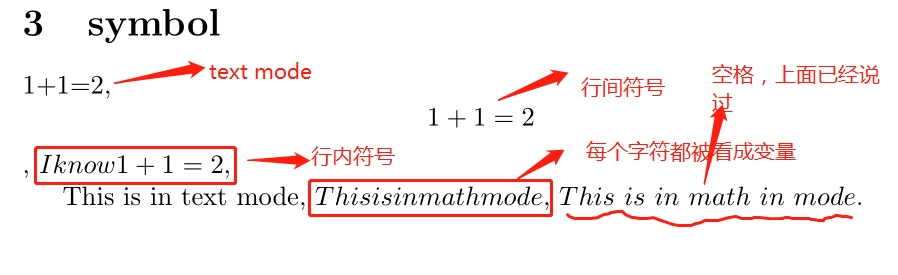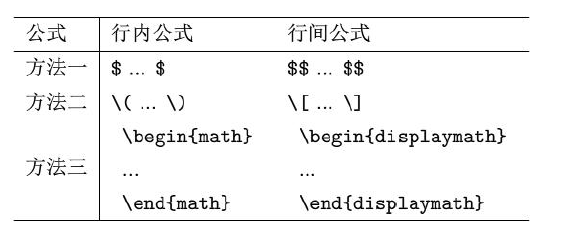6.输入函数

$\frac{10}{11}, \sqrt{x^2+1}, \ldots, \sin x, \cos x.$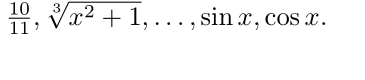$\frac{10}{11},$ $\sqrt{x^2+1},$ $\ldots,$ $\sin x,$ $\cos x.$


  $\sum_{n=1}^{\infty}$

$$\sum_{n=1}^{\infty}$$

$$\lim_{n \to \infty}(1+\frac{1}{n})^n = e,$$
$$\int_{-\infty}^{+\infty}\frac{\sin x}{x} \mathrm{d}x=I$$

$\alpha,\beta,\gamma,\delta\epsilon\varepsilon\xi\pi\rho\sigma\eta\theta\phi\varphi\omega$

$\Gamma\Phi$

$|A|,\|a\|,\vec{a},\overleftarrow{AB},\tilde{x},\widetilde{xyz},sin,\mathrm{sin}$

$\mathbb{AB},\mathbf{abAB}$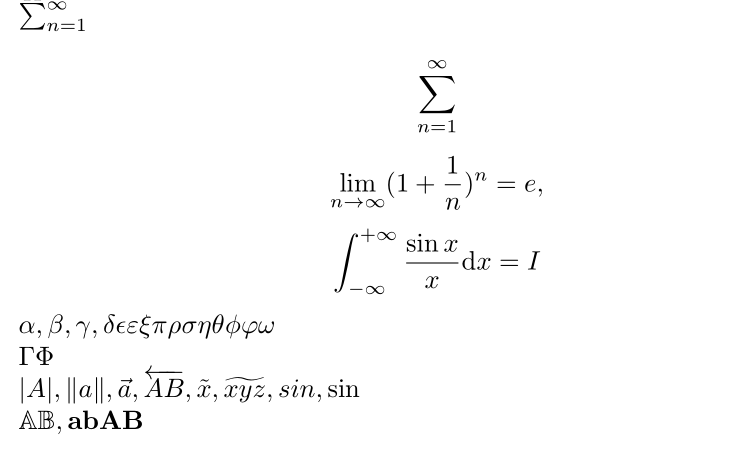（1）第一行是行内公式和第二行的行间公式是不一样的，这是出于文本美观的目的。
（2）\mathrm{d}那儿是为了和x不同，因为d是直立的而不是变量，而x是变量，这里mathrm=math roman
（3）剩下的看到gamma等希腊字母第一个字母大写则表示输出的文本是大写，这里值得注意的是：alpha,beta,varepsilon等是没有大写形式的，因为其大写形式就是ABE，不能输入\Alpha,\Beta…
（4）mathbb=math blackboard, mathbf=math boldface

7.矩阵和大括号

  \begin{equation}
\left(
\begin{array}{ccc}
a_{11}& a_{12} & a_{13} \\
a_{21} & a_{22} & a_{23} \\
a_{31} & a_{32} & a_{33}
\end{array}
\right)
\end{equation}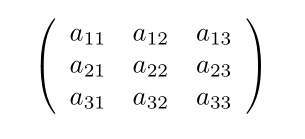\begin{equation}
f=
\left(
\begin{array}{ccc}
a_{11}& a_{12} & a_{13} \\
a_{21} & a_{22} & a_{23} \\
a_{31} & a_{32} & a_{33}
\end{array}
\right)
\end{equation}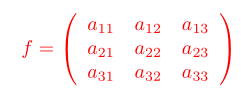（这里不要纠结颜色，因为我都改为红色了）
（1）以上begin{equation}开始了一个公式环境，在这个环境中可以直接输入变量、函数等而不需要加$符号，上面等同于  $$\left( \begin{array}{ccc} a_{11}& a_{12} & a_{13} \\ a_{21} & a_{22} & a_{23} \\ a_{31} & a_{32} & a_{33} \end{array} \right)$$  （2）\left( and \right) are left bracket and right bracket. 能够通过括号内的内容自动改变括号大小。所以上面6中的第三行应该改为： $$\lim_{n \to +\infty}\left(1+\frac{1}{n}\right)^n=e$$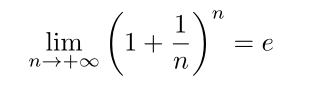（3）符号&是用来分隔列的，同一行不同列之间的元素用&分隔开 （4）｛ccc｝是array中必选参数，为center,center,center,表示三列都居中， （5）\是强制换行符号 8.分段函数 分段函数是在矩阵的基础之上的，比如如下的分段函数  \begin{equation} f(x)= \left\{ \begin{array}{cc} \int \mathrm{d}^3p\int_{0}^{\infty}\mathrm{d}x x^2 \frac{\delta(E-\sqrt{p^2+m^2})}{2E} , & x \geq 0 \\ 0,& x < 0 \end{array} \right. \end{equation}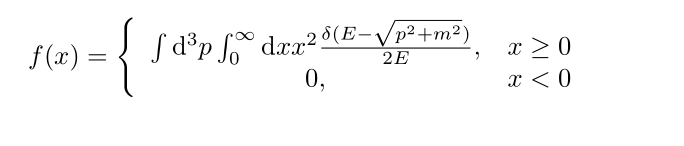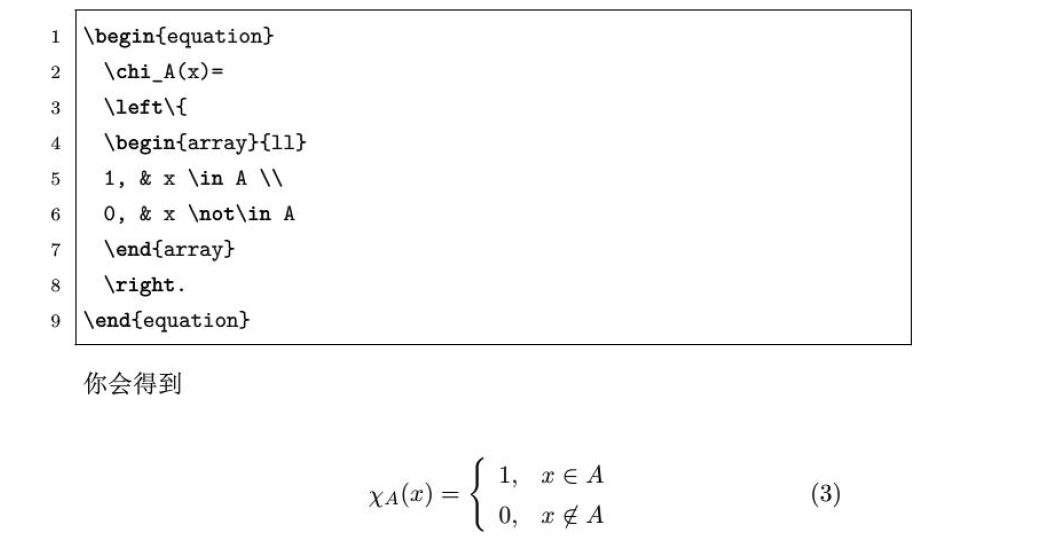（1）不需要右括号 （2）&的作用是使得列对齐 9.加载宏包doc文档 当我们使用一个宏包比如amsmath，想要得到说明文时可以如下操作： 在控制台上点击终端cmd窗口，输入tex xxx(xxx是宏包名，如amsmath)回车即可。 终端按钮如下图：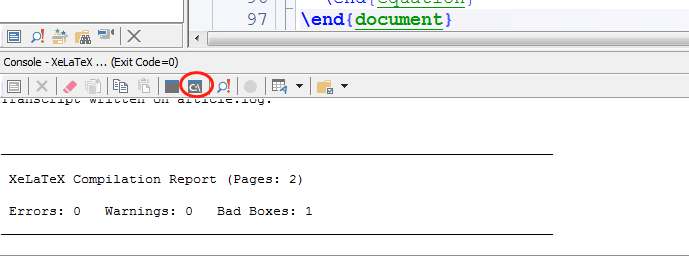10.输入中文以及宏包安装 输入中文需要用到ctex包，这是专门针对中文而设计的包，使用时在前面将 \document{article}  改为 \document[UTF8]{ctexart}  即可,（有的不需要加方括号中的内容），或者\document{article}不变，在其下加入一个宏包为 \usepackage[UTF8]{ctex}  此外，中文包还可以使用 \usepackage{CJK}  当然，前提是已经安装ctex宏包，通常情况都会自动安装好，如果没有安装可以如下（笔者用的是Tex live） 开始—tex live—tex live manager—找到ctex—点击安装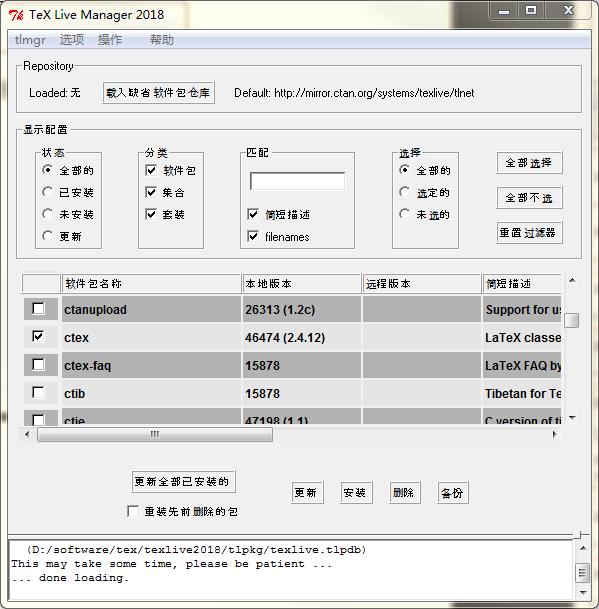值得注意的是：宏包已经都不需要网上下载安装，而是直接从库上安装缺少的宏包，方法就是上面的方法。 11.插入图片 插入图片可以引用命令： \ref{fig} or \autoref{fig}  前者只给图片序号，而后者为Figure + 序号 插入图片需要加载宏包 \usepackage{graphicx}  \usepackage{graphics}  建议前者。 插入图片的环境为：  \begin{figure}[ht] \centering \includegraphics[width=10cm]{hh} \caption{这个是图片}\label{bbb} \end{figure}  解释： （1）第一句begin{figure}代表开始插入图片，后面的[ht]是可选参数，表示here, top。如果去掉[ht]表示默认的htbp,分别表示“这里，顶部，底部，换一页”（here, top, bottom, page） （2）第二句是图片居中，否则自动靠左 （3）[width=10cm] (有些people不需要加cm，是已经设置单位是cm了，还可以有in英尺)，可选的参数还有如下（可参考DreamSeeking的文献）：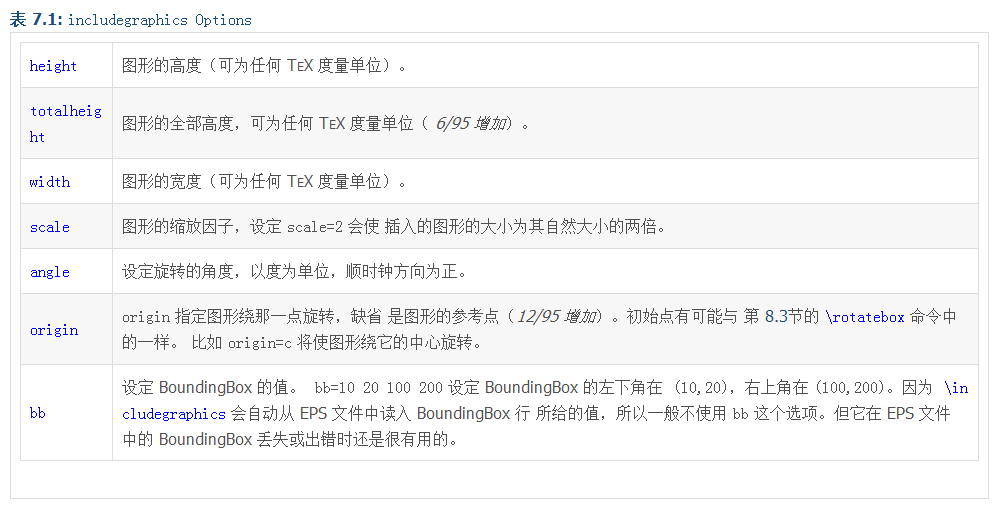第三行最后的｛hh｝表示插入的图片的名字，因此插入的图片一定要放在工作目录下，并命名为hh.xxx（xxx表示后缀，如png,jpg,eps等） 当然也有不在工作目录下的方法，这个google一下by yourself.在此不累赘。（好吧，让我再告诉你好了，其实就是使用绝对路径 \includegraphics[width=10cm]{D:/software/tex/hh.png}  当然，.png后缀可以不写）注意，绝对路径中是用的左斜杠“/”而不是右斜杠。 此外如果图片比较多，最好的方法是在当前工作路径下单独建一个文件夹，命名为fig.然后将图片都放进去，此后要使用里面的图片时只需要使用路径加载即可，这是相对路径方法，如下： \includegraphics[width=10cm]{fig/hh}  （4）caption｛这个是图片｝表示对图片的描述，将出现在图片的底下。label{bbb}表示对这张图片的标签，相当于给定了这张图片的名字。图片较多时，建议使用容易看懂的名字，如label{fig.sub.1}。这个label的作用是方便以后引用这个图时只需要用其标签就可以，比如 see,figure\ref{fig.sub.1} or \autoref{fig.sub.1}  结果就是：see,figure x（x表示刚刚插入的图的序号，如图3） （5）这样插入的图片是一个浮动图，也就是latex自动找到最好的位置来放置此图，而不是你代码设置的地方，想要得到不浮动的图可以将 \includegraphics[width=10cm]{fig/hh}  这句单独提取出来放置想要放的代码中即可，但这个时候是不能加 \caption{xxx}  这一句的，也就是没法加入标题。如果要给不浮动的图形加标题，那么可以看看nonfloat 包或者自己google(这回是朕是真的不打算给你找了)。 12.表格 表格制作可以参考这个 and 这个（点一下试试），后者是英文。 简单说明： 表格制作可以使用tabular，简单一个图如下：  \begin{tabular}{|c|c|} \hline % after \\: \hline or \cline{col1-col2} \cline{col3-col4} ... Name & score \\ \hline 小明 & 50 \\ 小华 & 45 \\ \hline \end{tabular}  结果如下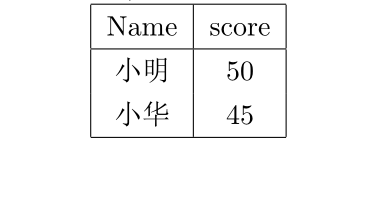讲解： （1）第一句是tabular表格的开始，参数{|c|c|}中的第一个|表示表格中的左边竖，第二、三个分别是中间和右边的竖。而c表示表格中的字体居中，l(left)和r(right)表示表格中字体靠左和靠右。可以尝试将｛|c|c|｝改为{c|c}即不要两边的竖线。 （2）\hline（horizontal line）表示画一条水平线 （3）%是注释，\是换行，\hline可以紧接在\后，如下表示： \\ \hline  会显得更紧凑。 当然，这个表格是不浮动的，和图片一样，如果想加入\caption的话，可以构成浮动的图片（但毕竟可以写标题了呀，想想还是赚的）。这个时候需要用到table环境。如下  \begin{table} \centering \begin{tabular}{|c|c|} \hline % after \\: \hline or \cline{col1-col2} \cline{col3-col4} ... Name & score \\ \hline 小明 & 50 \\ 小华 & 45 \\ \hline \end{tabular} \caption{这是一个表格} \end{table}  同样的\centering是表格居中，否则居左。其他都讲过了，很easy.最后可以使用caption宏包，这个需要自行google。 在表格中换行 比如两个表格(table)是要构成一个table的，想统一做一个说明，而不是每个table单独说明，此时需要将两个表格分开，这个时候可以用命令： \vspace{0.35cm} #表示上下空0.35cm的空间  比如 \begin{table} \centering Case 0: 95+150 \begin{tabular}{|c|c|c|c|c|c|} \hline$r$&$\sigma_r(\sigma_F=1)$&$\sigma_r(\sigma_F=0.1)$&$\sigma_r(\sigma_F=0.01)$&$N_{95}$&$N_{150}$\\ \hline 0.01 & 0.01094 & 0.00228 & 0.00106 & 8701 & 1299 \\ 0 & 0.01084 & 0.00216 & 0.00091 & 8701 & 1299 \\ \hline \end{tabular} \vspace{0.35cm} #空0.35cm Case 1: 41+95+150 \begin{tabular}{|c|c|c|c|c|c|} \hline$r$&$\sigma_r(\sigma_F=1)$&$\sigma_r(\sigma_F=0.1)$&$\sigma_r(\sigma_F=0.01)$&$N_{95}$&$N_{150}$\\ \hline 0.01 & 0.00787 & 0.00203 & 0.00106 & 8701 & 1299 \\ 0 & 0.01084 & 0.00216 & 0.00091 & 8701 & 1299 \\ \hline \end{tabular} \caption{table 1}\label{anser1} \end{table}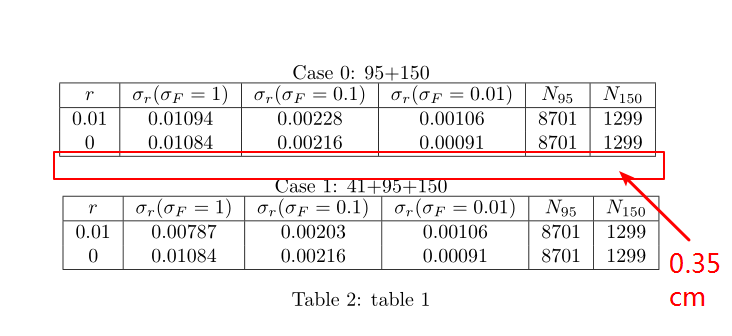合并行列 1,合并行 使用 \multirow{n}*{text} #n表示要合并的行数，*是必须的，否则无法编译成功，text是此行中要输入的内容  注意，使用\multirow一定要在导言区，也就是在最开头使用包\usepackage{multirow} 举例如下： % !Mode::"TeX:UTF-8" \documentclass{article} \usepackage[UTF8]{ctex} \usepackage{listings} \usepackage{amsthm} \usepackage{amsmath} \usepackage{amssymb} \usepackage{graphicx} \usepackage{hyperref} \usepackage[table]{xcolor} \usepackage{fancyhdr} \usepackage{lastpage} \usepackage{pythonhighlight} \pagestyle{fancy} \usepackage{enumitem} \setlist[trivlist]{topsep=\baselineskip} \usepackage{multirow} %这里以上都是导言区 \begin{document} \begin{tabular}{|c|c|c|} \hline \multirow{3}*{朕} & 尚书省 & 工部 刑部 兵部 礼部 户部 吏部 \\ \cline{2-3} & 中书省 & ... \\ \cline{2-3} & 门下省 & ... \\ \hline \end{tabular} \end{document}  得到如下图：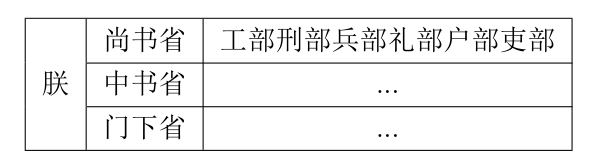上面中 \cline{a-b}表示从第a列画一条到第b列的线，与\hline的区别在于可以指定线的起始位置。 2,合并列 使用 \multicolumn{n}{x}{text} #n为合并列的数量，x为字体的排版样式，比如r,l,c分别表示右对齐，左对齐，居中，text为内容  例子 \begin{document} \begin{tabular}{|c|c|} \hline \multicolumn{2}{|c|}{成绩} \\ \hline 语文 & 数学 \\ 98 & 100 \\ \hline \end{tabular} \end{document}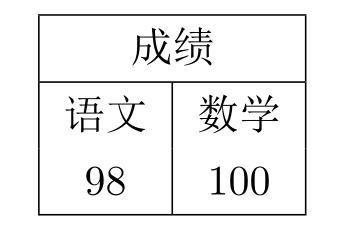3,行列都存在 \begin{document} \begin{tabular}{|c|r|r|} \hline \multirow{2}*{姓名} & \multicolumn{2}{c|}{成绩} \\ \cline{2-3} & 语文 & 数学 \\ \hline 小明 & 60 & 55 \\ \hline \end{tabular} \end{document}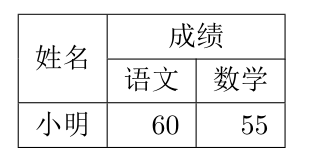表格下标注释和字体大小设置 给定一个表格后，表下可能会有表格的介绍，而介绍的字体通常比文章字体小，可以用 \small{}   \begin{table} \centering \rowcolors[\hline]{2}{orange}{red!30!yellow} \begin{tabular}{|c|c|} \rowcolor{red!20} Name & score \\ 小明 & 50 \\ 小华 & 45 \\ \hline \end{tabular} \caption{\small{This is a simple table for testing}} \end{table}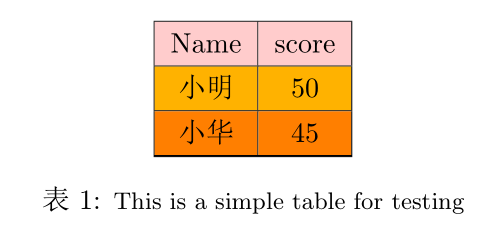设置表格（字体）大小 同样，可以通过\small来修改，但是放置的位置和上面不同，而是放在\begin{table}之后，如：  \begin{table} \centering \small #改变表格字体大小，同时表格大小也会变 \rowcolors[\hline]{2}{orange}{red!30!yellow} \begin{tabular}{|c|c|} \rowcolor{red!20} Name & score \\ 小明 & 50 \\ 小华 & 45 \\ \hline \end{tabular} \caption{\small{This is a simple table for testing}} \end{table}  此外还有  \tiny \scriptsize \footnotesize \small \normalsize \large \Large \LARGE \huge \Huge  彩色表格和字体 彩色表格 彩色表格需要用到宏包 \usepackage{xcolor}  没有需要按照上面的方法先安装。因为是针对表格，所以需要支持表格，使用时要如下： \usepackage[table]{xcolor}  上面的例子可以加入彩色如下： ... \usepackage[table]{xcolor} ... ... \begin{table} \centering \rowcolors[\hline]{2}{orange}{red!30!yellow} \begin{tabular}{|c|c|} \rowcolor{red!20} Name & score \\ 小明 & 50 \\ 小华 & 45 \\ \hline \end{tabular} \caption{这是一个表格} \end{table}  结果为：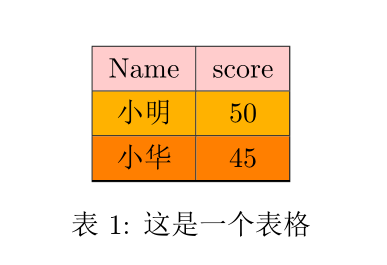说明： （1）第一行使用宏包xcolor，并支持table （2）  \rowcolors[\hline]{2}{orange}{red!30!yellow}  这里是表示行颜色的设置，\hline表示每行都加横线，后面表示从第二行开始奇数行用orange颜色，偶数行用30%红色和30%黄色的混合，也可以写成{red!30yellow!30} （3）因为我们是从第二行开始加颜色，所以第一行要单独加颜色，如下 \rowcolor{red!20}  如果没有则是白色。如果想要单独每行自行设置，也可以如下单独每行设置颜色  \begin{table} \centering \begin{tabular}{|c|c|} \hline \rowcolor{red!20} Name & score \\ \hline \rowcolor{yellow} 小明 & 50 \\ \hline \rowcolor{orange} 小华 & 45 \\ \hline \end{tabular} \caption{这是一个表格} \end{table}  彩色字体 同样是xcolor包 {\color{violet}{哇，这是我的第一个 \LaTeX{} Article!}}这一行是通过一个大花括号括起来的（即最左和最后的括号），如果没有则整篇文章都是这种颜色的字体，括起来后就只有这一句话是这个颜色。 更多颜色设置见官网 and it’s pdf article 13.交叉引用（corss-reference） 关于引用部分上面已经提及了一些，上面11的（4）我们用的是\ref {xxx}这个命令。这个命令有一个缺点是它只给出图、定理等的序号，比如“如图2所示”当我们使用\ref时需要写成： 如图\ref{xxx}所示  当我们并不知道这个\ref｛xxx｝是图还是表格还是定理等等的时候就比较麻烦，或者我们不想输入“图”，“表格”这样的字眼，这时可以使用宏包（同样，如果没有宏包先按照上面的方法安装） \usepackage{hyperref}  然后将\ref {xxx}改为\autoref{xxx},e.g. ...... \usepackage{hyperref} ... ... \begin{equation} f(x)= \left\{ \begin{array}{cc} \int \mathrm{d}^3p\int_{0}^{\infty}\mathrm{d}x x^2 \frac{\delta(E-\sqrt{p^2+m^2})}{2E} , & x \geq 0 \\ 0,& x < 0 \end{array} \right. \label{myequation} \end{equation} We all know \autoref{myequation} is a important equation  we can get: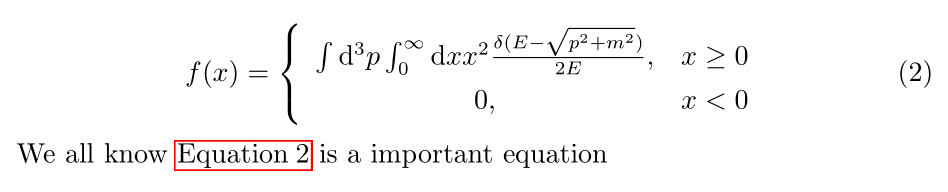可以看到自动加了equation这个词，而且是红色方框，点击可以直接跳到公式2. 14.文章布局 （1）页面大小 使用tex得到的pdf很窄，左右空的地方很大，如果我们想让它跟微软中的word一样的页面，可以在导言部分加入以下命令 \usepackage[top=2.54cm, bottom=2.54cm, left=3.18cm,right=3.18cm]{geometry}  （2）页眉页脚 参考文献见这里 使用宏包 \usepackage{fancyhdr}  在导言部分加入 \usepackage{fancyhdr} \pagestyle{fancy} \lhead{wudl} \chead{$wudl'stutorial article$} \rhead{\thesection} \cfoot{\thepage}  上面的结果是，页眉左上边是wudl，中间是chead中的句子，右上边是章节，页脚是页码。实际还有很多可选参数，自行查找需要的。 当然上面的页码是“1”“2”“3”这样的数，如果想要A of B,即第A页共B页，则要使用lastpage宏包。 如下： \usepackage{fancyhdr} \usepackage{lastpage} \pagestyle{fancy} \lhead{wudl} \chead{$wudl'stutorial article\$}
\cfoot{Page \thepage of \pageref{LastPage}}（3）列举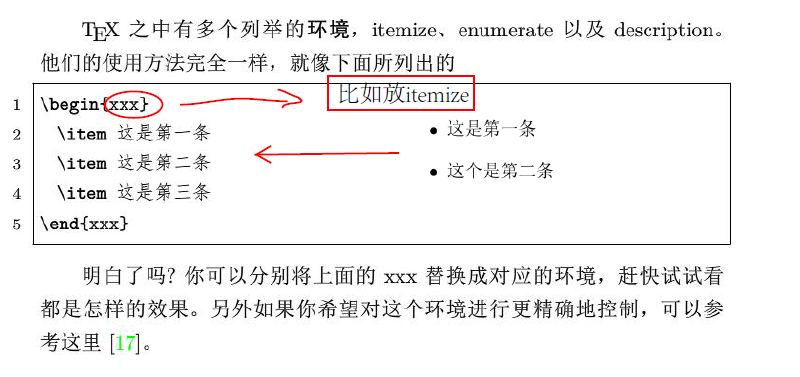（4）参考文献

A.手动输入

  As is stated in \cite{bibitem1} blahblah\dots
\begin{thebibliography}{9}

\bibitem{bibitem1}

\bibitem{bibitem2}
blahblah(ditto)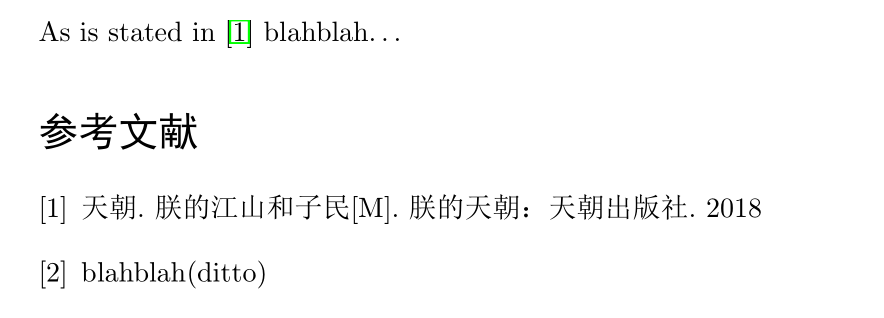15.超链接

hyperref


16.插入代码

%导言部分
...
...
\usepackage{listings}              %加入宏包
\lstset{language=Python}           %Python语言
\lstset{breaklines}                %自动将长的代码换行排版
\lstset{extendedchars=false}       %解决代码跨页时，章节标题，页眉等汉字不显示的问题
...
...
%需要插入代码的地方
\begin{lstlisting}

\end{lstlisting}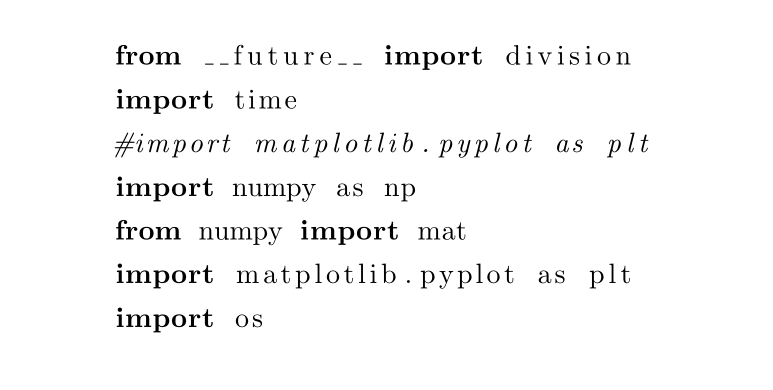\usepackage{listings}
\usepackage{color}

\definecolor{dkgreen}{rgb}{0,0.6,0}
\definecolor{gray}{rgb}{0.5,0.5,0.5}
\definecolor{mauve}{rgb}{0.58,0,0.82}

\lstset{ %
language=Octave,                % the language of the code
basicstyle=\footnotesize,           % the size of the fonts that are used for the code
numbers=left,                   % where to put the line-numbers
numberstyle=\tiny\color{gray},  % the style that is used for the line-numbers
stepnumber=2,                   % the step between two line-numbers. If it's 1, each line
% will be numbered
numbersep=5pt,                  % how far the line-numbers are from the code
backgroundcolor=\color{white},      % choose the background color. You must add \usepackage{color}
showspaces=false,               % show spaces adding particular underscores
showstringspaces=false,         % underline spaces within strings
showtabs=false,                 % show tabs within strings adding particular underscores
frame=single,                   % adds a frame around the code
rulecolor=\color{black},        % if not set, the frame-color may be changed on line-breaks within not-black text (e.g. commens (green here))
tabsize=2,                      % sets default tabsize to 2 spaces
captionpos=b,                   % sets the caption-position to bottom
breaklines=true,                % sets automatic line breaking
breakatwhitespace=false,        % sets if automatic breaks should only happen at whitespace
title=\lstname,                   % show the filename of files included with \lstinputlisting;
% also try caption instead of title
keywordstyle=\color{blue},          % keyword style
stringstyle=\color{mauve},         % string literal style
morekeywords={*,...}               % if you want to add more keywords to the set
}


...
...
\usepackage{graphicx}
\usepackage{pythonhighlight}
...
...
\inputpython{test.py}{1}{41}#导入python文件test.py第一行到第41行
...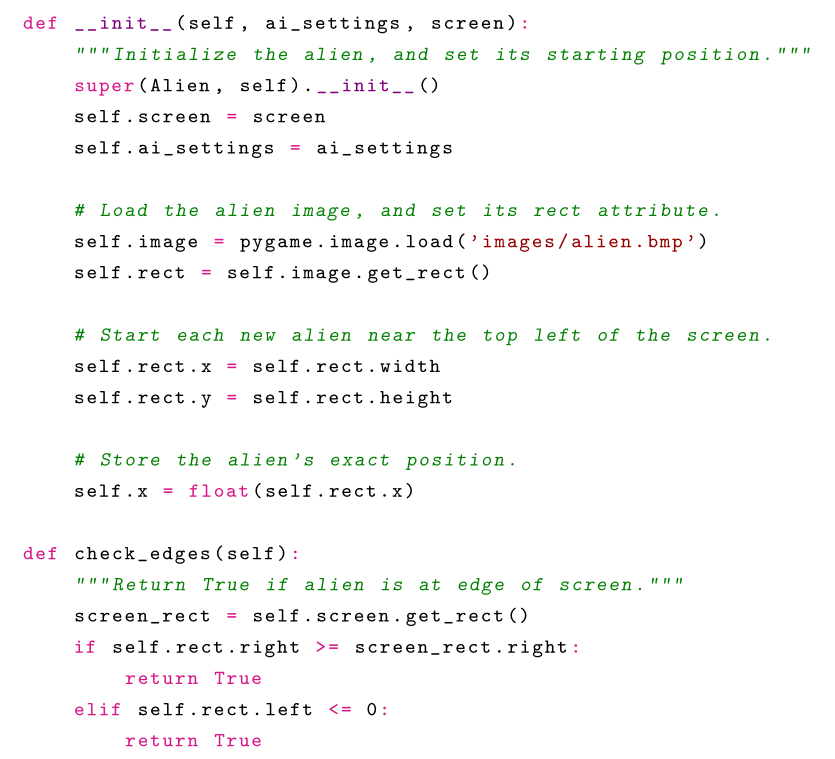\begin{xxx}

\end{xxx}


  \begin{python}
#
from pyx import *
def f():
g = graph.graphxy(width=8)
g.plot(graph.data.function("y(x)=sin(x)/x", min=-15, max=15))
g.writePDFfile("function")
print r'\includegraphics{function}'
return f
\end{python}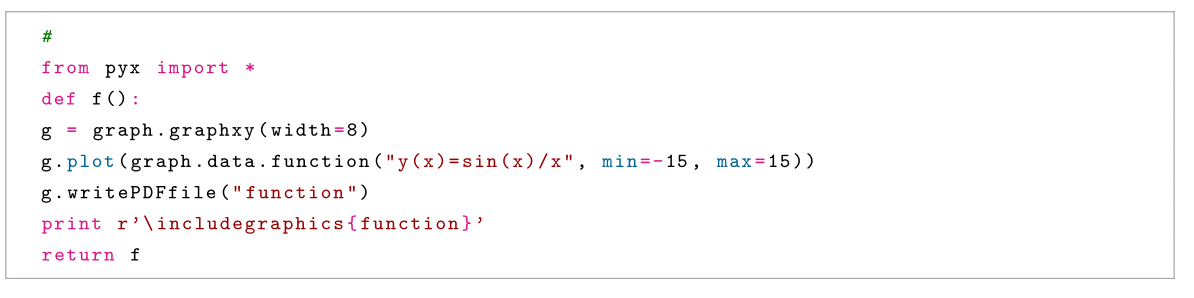17.目录生成

\tableofcontents


\documentclass{article}
\usepackage{hyperref}
\begin{document}
\tableofcontents
\section{A section}
This is a section
\paragraph{A paragraph}
This is a paragraph
\subsection{A subsection}
This is a subsection
\end{document}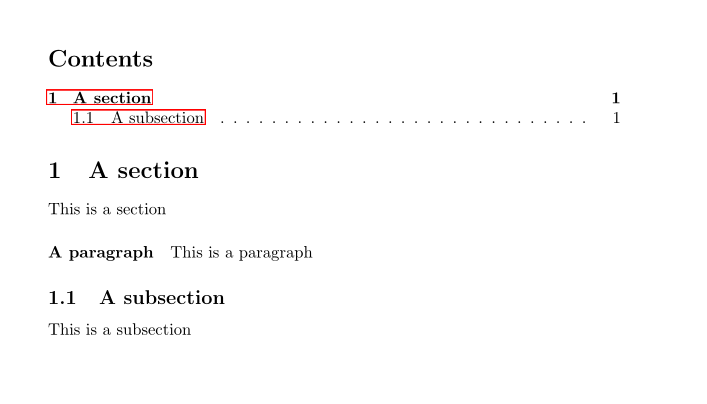\usepackage{hyerref}


18.数学公式对齐

\begin{equation}\label{information gain}
\begin{aligned}
Gain(X,Y)&=H(Y)-H(Y|X)\\
&=H(X)-H(X|Y)\\
&=H(X)+H(Y)-H(X,Y)
\end{aligned}
\end{equation}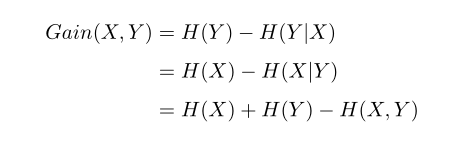\begin{equation}\label{lagrangian}
\begin{aligned}
w_{ij}&=\frac{1}{(N_i+R_i)(N_j+R_j)\frac{1}{2}(1+\delta_{ij})}\\
Var[\mathbb{\hat{C}}_\ell]&=\frac{2}{2\ell+1}\left(\sum_{i,j,j\ge i}\frac{1}{(N_i+R_i)(N_j+R_j)\frac{1}{2}(1+\delta_{ij})}\right)^{-1}
\end{aligned}
\end{equation}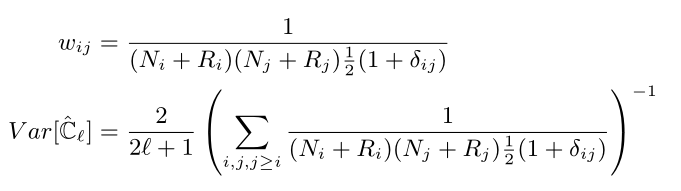19. 段落空行, 换行和首部缩进

A： Hello, how are you?
B： Hi, i'm fine.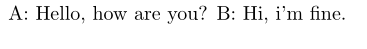A： Hello, how are you?\\
B： Hi, i'm fine.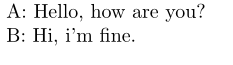A： Hello, how are you?

B： Hi, i'm fine.